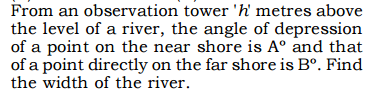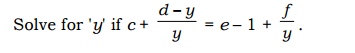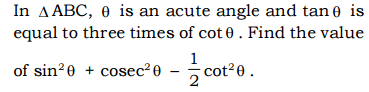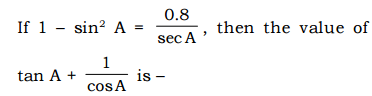## Maths Quiz for SSC CGL Tier-2 with Explanation

We have already provided some Maths Quizzes for SSC CGL Exam. If you want to check those quizzes, click on below given links:

# Maths Quiz for SSC CGL Tier-2

• #### 1.1. 0.278
2. 0.66
3. 0.5
4. 0.25
##### Answer And Explanation
Answer: Option C
Explanation:
Note:

# Maths Quiz for SSC CGL Tier-2

• #### 2.1. h (Cot B + Cot A)
2. h (Tan B + Tan A)
3. h (Tan A - Tan B)
4. h (Cot B- Cot A)
##### Answer And Explanation
Answer: Option D
Explanation:
Note:

# Maths Quiz for SSC CGL Tier-2

• #### 3. A right pyramid stands on a rectangular base whose sides are 24 cm and 18 cm and on the shorter side, the slant height is 17 cm. Find the volume of the pyramid.

1. 1735.5 cubic centi metre
2. 17.533 cubic centi metre
3. 1733.5 cubic centi metre
4. 17.335 cubic centi metre
##### Answer And Explanation
Answer: Option C
Explanation:
Note:

# Maths Quiz for SSC CGL Tier-2

• #### 4.1.  K + l + m + n
2.  K + n + √ m - n
3. +-[√ K + l + √ m - n
4.  K + m + √ l + n
##### Answer And Explanation
Answer: Option C
Explanation:
Note:

# Maths Quiz for SSC CGL Tier-2

• #### 5.1. (d -f)/ (e +c)
2. (d -f)/ (e - c)
3. (d + f)/ (e - c)
4. (d + f)/ (e + c)
##### Answer And Explanation
Answer: Option B
Explanation:
Note:

# Maths Quiz for SSC CGL Tier-2

• #### 6.1. 23/12
2. 23/9
3. 23/11
4. 23/8
##### Answer And Explanation
Answer: Option A
Explanation:
Note:

# Maths Quiz for SSC CGL Tier-2

• #### 7.1. 14.26 metre square
2. 8.38 metre square
3. 9.46 metre square
4. 12.26 metre square
##### Answer And Explanation
Answer: Option A
Explanation:
Note:

# Maths Quiz for SSC CGL Tier-2

• #### 8. A man rides at the rate of 300 Metres per minute and stops for 6 minutes to change horses at the end of every sixth Kilometre. Then to travel a distance of 84 km, the time taken by the man is

1. 3 hours 15 minutes
2. 6 hours 17 minutes
3. 5 hours 18 minutes
4. 2 hours 17 minutes
##### Answer And Explanation
Answer: Option C
Explanation:
Note: Speed of horse rider = 350 metre/minute = 21 km/h

84/6 = 14 . It means, he will change the horse 13 times.

Time taken in changing the horses = 13 x 6 = 78 minutes
Time taken in covering 84 km = 4 hours
Total time = 4 hours + 78 minutes
= 5 hours 18 minutes

# Maths Quiz for SSC CGL Tier-2

• #### 9.1. 2/ (1+a)
2. 2/(1- a)
3. 1/(1+a)
4. None of these

# Maths Quiz for SSC CGL Tier-2

• #### 10.1. 0
2. 1/2
3. 2
4. 1.5
##### Answer And Explanation
Answer: Option C
Explanation:
Note:

#### Post a Comment

Leave your comment but never use bad words otherwise you will be blocked.